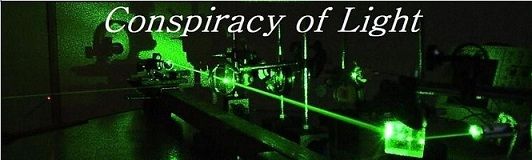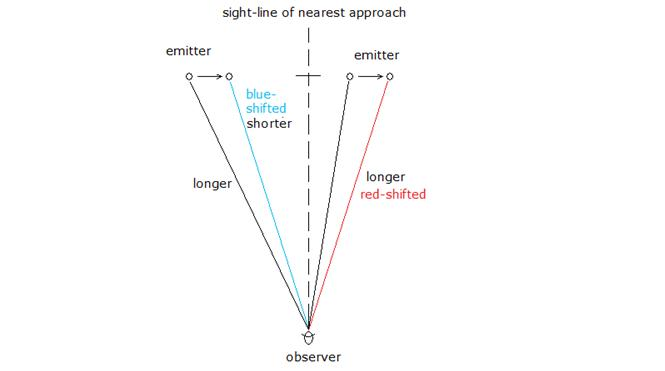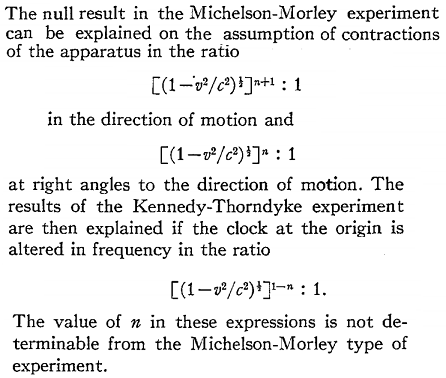The search for  new physics.

Transverse Doppler Effect:

Although one would not ordinarily expect a Doppler shift of light for an object moving transversally to the observer, it stands to reason that for any object moving transversally to an observer, a line may be drawn between that object and the observer, and it can be shown that this line will shorten if the objects is moving transversally such that the object is approaching a sight line forming the nearest approach of the object. Similarly, the object will appear red-shifted as it moves transversally away from this similar sight line.An approaching object will appear blue-shifted by an amount equal to the effective velocity at which the object is approaching along the sight line. A receding object will appear red-shifted by an amount equal to the effective velocity at which the object is receding from the observer along its sight line. This is the Classical Transverse Doppler shift.

Special Relativity (1907) adds an additional amount of red-shift, this amount being in all cases:When the object is at the nearest approach (perpendicular to the observer), the red-shift is by exactly this amount. Herbert Ives proved this it in his paper 1938 and 1941 papers, in a set of experiments which have become known as the Ives-Stillwell experiments. It is interesting to note however that Ives was never seeking to prove an element of Einstein's theory with this work. Rather, Ives sought instead to confirm the predictions of Larmor and Lorentz (i.e. Lorentz ether theory) as is stated repeatedly in his papers.

Ives outlined his thesis in his 1937 paper "The Doppler Effect Considered in Relation to the Michelson-Morley Experiment". In this paper he notes the null result of both the Michelson-Morley (MM) and Kennedy-Thorndike (KT) experiments, which were historically explained by Fitzgerald and Lorentz by the introduction of a physical Lorentz contraction in the direction of the motion of the apparatus. However, Ives was unsatisfied by the idea of experimentally supporting the contraction hypothesis by a negative result. The Ives-Stillwell experiment was conceived as an experiment to demonstrate the existence of the physical Lorentz contraction by a positive experimental result (and by this he did not mean the Lorentz contraction hypothesis of Einstein, which has a different, non-physical meaning). Ives considered that the MM and KT experiments could be explained by a series of possibilities with respect to the contractions of the moving apparatus and the alteration of the frequency of the moving clock,as shown in the figure below.So Ives's two objectives were to:

1) Prove that the physical Lorentz contraction is real, and

2) determine the value of n in the equations above.

This was because he felt that the Doppler effect exhibited by the transverse emission of the moving canal ray particles would be modulated by the physical Lorentz contraction. As Ives states in his 1937 paper:

“ In this invariance the Doppler effect phenomena thus become similar to the Michelson-Morley phenomena. There is however a vital difference in the character of this invariance. In the case of the Michelson Morley experiment the function of the term [(1-v^2/c^2))^ ½] ^n+1 is to make the effect not only invariant but null. That is, the predicted behavior corresponds to the “classical” case for the apparatus stationary in the ether.  In the Doppler effect, the result predicted is invariant, but different from the classical case for the observer stationary in the ether, as shown by comparing Eqs. (12), (13) and (14), with equations (6), (7) and (8), with Vo  put equal to zero. Experiments with canal rays, of the sort here assumed, should therefore be capable of furnishing positive optical evidence for the validity of the contractions postulated by Fitzgerald, Larmor and Lorentz.”

From reading Ives rationale it becomes clear why this canal ray red-shift is a direct consequence of the Doppler effect modulated by the Lorentz contraction and not necessarily of an Einsteinian time dilation. This can be summarized as follows:

- a moving particle source is a clock, and since it is moving relative to the stationary ether of Lorentz for which the speed of light is constant in this preferred frame only, its clock speed will mechanically slow as a consequence of the increased distances light has to move due to its motion. As Ives says, this phenomenon is similar to the MM phenomena, and this model is elaborated on diagrammitically in  Time dilation - and how it could be an Illusion?  The result of Ives 1938 and 1941 experiment was that positive evidence for this physical Lorentz contraction was found, and the value of n was determined to be 0.

The ultimate consequence is that the moving particle clock source will then count at a rate:

= fmoving = fstationary x (1-v2/c2)1/2. i.e. slower.

The frequency of any light emitted from this moving source will then appear lower to any observer, when other shifts due to the classical transverse Doppler effects are discounted (i.e. when the source is exactly perpendicular to the observer).

This is why Ives, rightly so, says that his experiment can be seen to validate the work of Lorentz and Larmor - because this confirms the mechanical slowing of clocks by a mechanism involving the Lorentz contraction. The theoretical basis for this observation predates Einstein in that it is derived from the earlier work of Fitzgerald and in the explanations for the null result of the Michelson-Morley experiment. As Ives points out, it is actually a positive result in favor of the physical Lorentz contraction hypothesis.

References:

1)  Einstein, A., (1907) "Über die Möglichkeit einer neuen Prüfung des Relativitätsprinzips", Annalen der Physik SER.4, no.23

2) Ives, Herbert, “The Doppler Effect Considered in Relation to the Michelson-Morley Experiment.” J.O.S.A. Vol.27, 1937, p.389

3) Ives, Herbert, “ An Experimental Study of the Rate of a Moving Atomic Clock.”  J.O.S.A. Vol. 28, 1938, P. 215.

4) Ives, Herbert, “ An Experimental Study of the Rate of a Moving Atomic Clock.” II J.O.S.A. Vol. 31, 1941, P. 369.